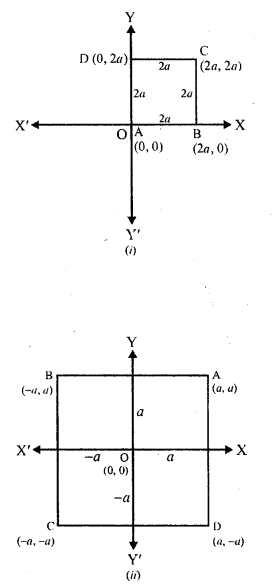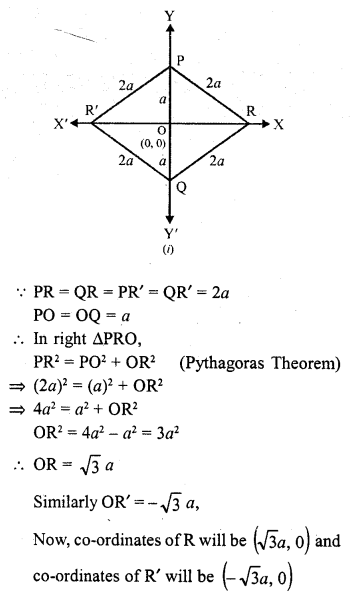# RD Sharma Class 10 Solutions Chapter 6 Co-ordinate Geometry Ex 6.1

## RD Sharma Class 10 Solutions Chapter 6 Co-ordinate Geometry Ex 6.1

These Solutions are part of RD Sharma Class 10 Solutions. Here we have given RD Sharma Class 10 Solutions Chapter 6 Co-ordinate Geometry Ex 6.1

Other Exercises

Question 1.
On which axis do the following points lie?
(i) P (5, 0)
(ii) Q (0 – 2)
(iii) R (-4, 0)
(iv) S (0, 5)
Solution:
(i) P (5, 0)
Its ordinate or y-axis is 0. It lies on x-axis
(ii) Q (0 – 2)
Its abscissa or x-axis is 0. It lies on y-axis
(iii) R (-4, 0)
Its ordinate is 0 It lies on x-axis
(iv) S (0, 5)
Its abscissa is 0. It lies on y-axis

Question 2.
Let ABCD be a square of side 2a. Find the coordinates of the vertices of this square when
(i) A coincides with the origin and AB and AD are along OX and OY respectively.
(ii) The centre of the square is at the-origin and coordinate axes are parallel to the sides AB and AD respectively.
Solution:
ABCD is a square whose side is 2a
(i) A coincides with origin (0, 0)
AB and AD are along OX and OY respectively
Co-ordinates of A are (0, 0), of B are (2a, 0) of C are (2a, 2a) and of D are (0, 2a)
(ii) The centre of the square is at the origin (0, 0) and co-ordinates axes are parallel to the sides AB and AD respectively.
Then the co-ordinates of A are (a, a) of B are (-a, a), of C are (-a, -a) and of D are (a, -a) as shown in the figure given below :Question 3.
The base PQ of two equilateral triangles PQR and PQR’ with side 2a lies along y- axis such.that the mid-point of PQ is at the origin. Find the coordinates of the vertices R and R’ of the triangles.
Solution:
∆PQR and PQR’ are equilateral triangles with side 2a each and base PQ and mid of point of PQ is 0 (0, 0) and PQ lies along y-axisHope given RD Sharma Class 10 Solutions Chapter 6 Co-ordinate Geometry Ex 6.1 are helpful to complete your math homework.

If you have any doubts, please comment below. Learn Insta try to provide online math tutoring for you.